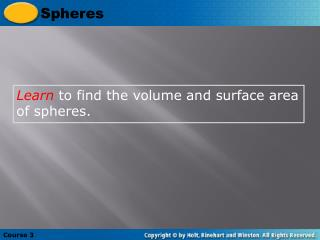DownloadDownload PresentationLearn to find the volume and surface area of spheres.

# Learn to find the volume and surface area of spheres.

Télécharger la présentation## Learn to find the volume and surface area of spheres.

- - - - - - - - - - - - - - - - - - - - - - - - - - - E N D - - - - - - - - - - - - - - - - - - - - - - - - - - -
##### Presentation Transcript

1. Spheres Course 3 Learn to find the volume and surface area of spheres.

2. Spheres Course 3 Insert Lesson Title Here Vocabulary sphere hemisphere great circle

3. Spheres Course 3 A sphere is the set of points in three dimensions that are a fixed distance from a given point, the center. A plane that intersects a sphere through its center divides the two halves or hemispheres. The edge of a hemisphereis agreat circle.

4. Theorem 12.11: Surface Area of a Sphere • The surface area of a sphere with radius r is S = 4r2.

5. Ex. 1: Finding the Surface Area of a Sphere • Find the surface area. When the radius doubles, does the surface area double?

6. S = 4r2 = 422 = 16 in.2 S = 4r2 = 442 = 64 in.2 The surface area of the sphere in part (b) is four times greater than the surface area of the sphere in part (a) because 16 • 4 = 64 So, when the radius of a sphere doubles, the surface area DOES NOT double.

7. More . . . • If a plane intersects a sphere, the intersection is either a single point or a circle. If the plane contains the center of the sphere, then the intersection is a great circle of the sphere. Every great circle of a sphere separates a sphere into two congruent halves called hemispheres.

8. Ex. 2: Using a Great Circle • The circumference of a great circle of a sphere is 13.8 feet. What is the surface area of the sphere?

9. Solution: Begin by finding the radius of the sphere. C = 2r 13.8 = 2r 13.8 2r 6.9 = r = r

10. Solution: Using a radius of 6.9 feet, the surface area is: S = 4r2 = 4(6.9)2 = 190.44 ft.2 So, the surface area of the sphere is 190.44  ft²

11. Theorem 12.12: Volume of a Sphere • The volume of a sphere with radius r is • V = 4r3. 3

12. Spheres 4 3 V = pr3 = p(9)3 4 3 Course 3 Additional Example 1: Finding the Volume of a Sphere Find the volume of a sphere with radius 9 cm, both in terms of p and to the nearest tenth of a unit. Volume of a sphere Substitute 9 for r. = 972p cm3 3,052.1 cm3

13. Spheres 4 3 V = pr3 Course 3 Try This: Example 1 Find the radius of a sphere given volume of a sphere is 113.1 m³. Find the circumference of the great circle given volume = 72pin³ Volume of a sphere

14. Spheres Course 3 Insert Lesson Title Here Lesson Quiz: Part 1 Find the volume of each sphere, both in terms of  and to the nearest tenth. Use 3.14 for p. 1.r = 4 ft 2.d = 6 m Find the surface area of each sphere, both in terms of  and to the nearest tenth. Use 3.14 for p. 85.3p ft3, 267.8 ft3 36p m3, 113.0 m3 1936p in2, 6079.0 in2 3.r = 22 in 4.d = 1.5 mi 2.25p mi2, 7.1 mi2

15. Spheres Course 3 Insert Lesson Title Here Lesson Quiz: Part 2 5.A basketball has a circumference of 29 in. To the nearest cubic inch, what is its volume? 412 in3